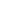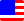Documents  >  Tutorial  >  Priority 4
Priority 4 operators

It describes the following operators with priority 4.

 * x * y Multiplication operator / x / y Division operator % x % y Modulo operator

They are binary arithmetic operators and perform arithmetic operations to the left and right operands.

The operand must be one of the int, long, or real classes. These classes can be mixed in the left and right operands.

Multiplication operator

Division operator

Modulo operator

Multiplication operator

Multiplication operator is an operator for multiplying the operands.

The class of the result depends on the class of the operand.

If there is the real class in the operand, the class of the result is real.

real r0 = 123.5 * 10.0; // The left is real and the right is real. Therefore, result is real.

real r1 = 123.5 * 10; // The left is real and the right is int. Therefore, result is real.

real r2 = 123.5 * 10L; // The left is real and right is long. Therefore, result is real.

If there is no real class in the operand and there is the long class, the class of the result is long.

long l0 = 123L * 10L; // The left is long and the right is long. Therefore, result is long.

long l1 = 123L * 10; // The left is long and the right is int. Therefore, result is long.

Otherwise the class of the result is int.

real i0 = 123 * 10; // The left is int and the right is int. Therefore, result is int.

An exception is thrown if the result exceeds the range of class values.

int max = 0.Max;

int result = max * 2; // Over flow. Exception will be thrown.

If operands return the proxy class, the entity of the proxy is automatically used. The result is the same as calling the Entity getter of the proxy class.

int i = 10;

real r = 10.5;

proxy pro = new proxy(i);

proxy pro2 = new proxy(r);

real r2 = pro2 * pro; // r2 is 105.

Division operator

Division operator is an operator for dividing the operands.

The class of the result depends on the class of the operand.

If there is the real class in the operand, the class of the result is real.

real r0 = 123.5 / 10.0; // The left is real and the right is real. Therefore, result is real.

real r1 = 123.5 / 10; // The left is real and the right is int. Therefore, result is real.

real r2 = 123.5 / 10L; // The left is real and right is long. Therefore, result is real.

If there is no real class in the operand and there is the long class, the class of the result is long.

long l0 = 123L / 10L; // The left is long and the right is long. Therefore, result is long.

long l1 = 123L / 10; // The left is long and the right is int. Therefore, result is long.

Otherwise the class of the result is int.

real i0 = 123 / 10; // The left is int and the right is int. Therefore, result is int.

If the result class is int or long, the remainder is truncated.

If division by zero, an exception is thrown.

If the value of the result exceeds the range of the class, an exception is thrown.

If operands return the proxy class, the entity of the proxy is automatically used. The result is the same as calling the Entity getter of the proxy class.

int i = 10;

real r = 10.5;

proxy pro = new proxy(i);

proxy pro2 = new proxy(r);

real r2 = pro2 / pro; // r2 is 1.

Modulo operator

Modulo operator is an operator that divides an operand to find the remainder.

The class of the result depends on the class of the operand.

If there is the real class in the operand, the class of the result is real.

real r0 = 123.5 % 10.0; // The left is real and the right is real. Therefore, result is real.

real r1 = 123.5 % 10; // The left is real and the right is int. Therefore, result is real.

real r2 = 123.5 % 10L; // The left is real and right is long. Therefore, result is real.

If there is no real class in the operand and there is the long class, the class of the result is long.

long l0 = 123L % 10L; // The left is long and the right is long. Therefore, result is long.

long l1 = 123L % 10; // The left is long and the right is int. Therefore, result is long.

Otherwise the class of the result is int.

real i0 = 123 % 10; // The left is int and the right is int. Therefore, result is int.

If division by zero, an exception is thrown.

If the value of the result exceeds the range of the class, an exception is thrown.

If operands return the proxy class, the entity of the proxy is automatically used. The result is the same as calling the Entity getter of the proxy class.

int i = 10;

real r = 10.5;

proxy pro = new proxy(i);

proxy pro2 = new proxy(r);

real r2 = pro2 % pro; // r2 is 0.5.